# Solution for submission 128953

A detailed solution for submission 128953 submitted for challenge IIT-M RL-ASSIGNMENT-2-TAXI

# What is the notebook about?¶

## Problem - Taxi Environment Algorithms¶

This problem deals with a taxi environment and stochastic actions. The tasks you have to do are:

• Implement Policy Iteration
• Implement Modified Policy Iteration
• Implement Value Iteration
• Implement Gauss Seidel Value Iteration
• Visualize the results
• Explain the results

## How to use this notebook? 📝¶

• This is a shared template and any edits you make here will not be saved.You should make a copy in your own drive. Click the "File" menu (top-left), then "Save a Copy in Drive". You will be working in your copy however you like.

• Update the config parameters. You can define the common variables here

Variable Description
AICROWD_DATASET_PATH Path to the file containing test data. This should be an absolute path.
AICROWD_RESULTS_DIR Path to write the output to.
AICROWD_ASSETS_DIR In case your notebook needs additional files (like model weights, etc.,), you can add them to a directory and specify the path to the directory here (please specify relative path). The contents of this directory will be sent to AIcrowd for evaluation.
AICROWD_API_KEY In order to submit your code to AIcrowd, you need to provide your account's API key. This key is available at https://www.aicrowd.com/participants/me

# Setup AIcrowd Utilities 🛠¶

We use this to bundle the files for submission and create a submission on AIcrowd. Do not edit this block.

In :
!pip install aicrowd-cli > /dev/null

ERROR: google-colab 1.0.0 has requirement requests~=2.23.0, but you'll have requests 2.25.1 which is incompatible.
ERROR: datascience 0.10.6 has requirement folium==0.2.1, but you'll have folium 0.8.3 which is incompatible.


# AIcrowd Runtime Configuration 🧷¶

Get login API key from https://www.aicrowd.com/participants/me

In :
import os

AICROWD_DATASET_PATH = os.getenv("DATASET_PATH", os.getcwd()+"/13d77bb0-b325-4e95-a03b-833eb6694acd_a2_taxi_inputs.zip")
AICROWD_RESULTS_DIR = os.getenv("OUTPUTS_DIR", "results")

In :


API Key valid
Saved API Key successfully!
13d77bb0-b325-4e95-a03b-833eb6694acd_a2_taxi_inputs.zip: 100% 31.2k/31.2k [00:00<00:00, 309kB/s]

In :
!unzip $AICROWD_DATASET_PATH  Archive: /content/13d77bb0-b325-4e95-a03b-833eb6694acd_a2_taxi_inputs.zip creating: inputs/ inflating: inputs/inputs_base.npy inflating: inputs/inputs_1.npy inflating: inputs/inputs_0.npy inflating: inputs/inputs_2.npy creating: targets/ inflating: targets/targets_2.npy inflating: targets/targets_0.npy inflating: targets/targets_1.npy inflating: targets/targets_base.npy  In : DATASET_DIR = 'inputs/'  ## Taxi Environment¶ Read the environment to understand the functions, but do not edit anything In : import numpy as np class TaxiEnv_HW2: def __init__(self, states, actions, probabilities, rewards, initial_policy): self.possible_states = states self._possible_actions = {st: ac for st, ac in zip(states, actions)} self._ride_probabilities = {st: pr for st, pr in zip(states, probabilities)} self._ride_rewards = {st: rw for st, rw in zip(states, rewards)} self.initial_policy = initial_policy self._verify() def _check_state(self, state): assert state in self.possible_states, "State %s is not a valid state" % state def _verify(self): """ Verify that data conditions are met: Number of actions matches shape of next state and actions Every probability distribution adds up to 1 """ ns = len(self.possible_states) for state in self.possible_states: ac = self._possible_actions[state] na = len(ac) rp = self._ride_probabilities[state] assert np.all(rp.shape == (na, ns)), "Probabilities shape mismatch" rr = self._ride_rewards[state] assert np.all(rr.shape == (na, ns)), "Rewards shape mismatch" assert np.allclose(rp.sum(axis=1), 1), "Probabilities don't add up to 1" def possible_actions(self, state): """ Return all possible actions from a given state """ self._check_state(state) return self._possible_actions[state] def ride_probabilities(self, state, action): """ Returns all possible ride probabilities from a state for a given action For every action a list with the returned with values in the same order as self.possible_states """ actions = self.possible_actions(state) ac_idx = actions.index(action) return self._ride_probabilities[state][ac_idx] def ride_rewards(self, state, action): actions = self.possible_actions(state) ac_idx = actions.index(action) return self._ride_rewards[state][ac_idx]  ## Example of Environment usage¶ In : def check_taxienv(): # These are the values as used in the pdf, but they may be changed during submission, so do not hardcode anything states = ['A', 'B', 'C'] actions = [['1','2','3'], ['1','2'], ['1','2','3']] probs = [np.array([[1/2, 1/4, 1/4], [1/16, 3/4, 3/16], [1/4, 1/8, 5/8]]), np.array([[1/2, 0, 1/2], [1/16, 7/8, 1/16]]), np.array([[1/4, 1/4, 1/2], [1/8, 3/4, 1/8], [3/4, 1/16, 3/16]]),] rewards = [np.array([[10, 4, 8], [ 8, 2, 4], [ 4, 6, 4]]), np.array([[14, 0, 18], [ 8, 16, 8]]), np.array([[10, 2, 8], [6, 4, 2], [4, 0, 8]]),] initial_policy = {'A': '1', 'B': '1', 'C': '1'} env = TaxiEnv_HW2(states, actions, probs, rewards, initial_policy) #print("All possible states", env.possible_states) #print("All possible actions from state B", env.possible_actions('B')) #print("Ride probabilities from state A with action 2", env.ride_probabilities('A', '2')) #print("Ride rewards from state C with action 3", env.ride_rewards('C', '3')) base_kwargs = {"states": states, "actions": actions, "probabilities": probs, "rewards": rewards, "initial_policy": initial_policy} return base_kwargs base_kwargs = check_taxienv() env = TaxiEnv_HW2(**base_kwargs)  ## Task 1 - Policy Iteration¶ Run policy iteration on the environment and generate the policy and expected reward In : # 1.1 Policy Iteration def policy_iteration(taxienv, gamma): # A list of all the states states = taxienv.possible_states # Initial values values = {s: 0 for s in states} # This is a dictionary of states to policies -> e.g {'A': '1', 'B': '2', 'C': '1'} policy = taxienv.initial_policy.copy() ## Begin code here # Hints - # Do not hardcode anything # Only the final result is required for the results # Put any extra data in "extra_info" dictonary for any plots etc # Use the helper functions taxienv.ride_rewards, taxienv.ride_probabilities, taxienv.possible_actions # For terminating condition use the condition exactly mentioned in the pdf done = 0 count_iters = 0 while done == 0: count_iters +=1 delta = 1 while delta>1e-8: delta = 0 future_value_array = np.array([values[i] for i in states]) for s in states: j_old = values[s] a = policy[s] ride_prob = taxienv.ride_probabilities(s,a) ride_rew = taxienv.ride_rewards(s,a) values[s] = np.sum(ride_prob*(ride_rew+gamma*future_value_array)) delta = max(delta,np.abs(values[s]-j_old)) done = 1 future_value_array = np.array([values[i] for i in states]) for s in states: old_action = policy[s] curr_max_value = '' curr_max_action = '' for action in taxienv.possible_actions(s): ride_rew = taxienv.ride_rewards(s,action) ride_prob = taxienv.ride_probabilities(s,action) action_value = np.sum(ride_prob*(ride_rew+gamma*future_value_array)) if curr_max_value == '': curr_max_action = action curr_max_value = action_value elif action_value >= curr_max_value: curr_max_value = action_value curr_max_action = action if curr_max_action !=old_action: done = 0 policy[s] = curr_max_action # Put your extra information needed for plots etc in this dictionary extra_info = {'iterations':count_iters} ## Do not edit below this line # Final results return {"Expected Reward": values, "Policy": policy}, extra_info  # Task 2 - Policy Iteration for multiple values of gamma¶ Ideally this code should run as is In : # 1.2 Policy Iteration with different values of gamma def run_policy_iteration(env): gamma_values = np.arange(5, 100, 5)/100 results, extra_info = {}, {} for gamma in gamma_values: results[gamma], extra_info[gamma] = policy_iteration(env, gamma) return results, extra_info results, extra_info = run_policy_iteration(env)  # Task 3 - Modifed Policy Iteration¶ Implement modified policy iteration (where Value iteration is done for fixed m number of steps) In : # 1.3 Modified Policy Iteration def modified_policy_iteration(taxienv, gamma, m): # A list of all the states states = taxienv.possible_states # Initial values values = {s: 0 for s in states} # This is a dictionary of states to policies -> e.g {'A': '1', 'B': '2', 'C': '1'} policy = taxienv.initial_policy.copy() ## Begin code here # Hints - # Do not hardcode anything # Only the final result is required for the results # Put any extra data in "extra_info" dictonary for any plots etc # Use the helper functions taxienv.ride_rewards, taxienv.ride_probabilities, taxienv.possible_actions # For terminating condition use the condition exactly mentioned in the pdf done = 0 while done == 0: delta = 1 for random_iterator in range(m): delta = 0 future_value_array = np.array([values[i] for i in states]) for s in states: j_old = values[s] a = policy[s] ride_prob = taxienv.ride_probabilities(s,a) ride_rew = taxienv.ride_rewards(s,a) values[s] = np.sum(ride_prob*(ride_rew+gamma*future_value_array)) delta = max(delta,np.abs(values[s]-j_old)) done = 1 future_value_array = np.array([values[i] for i in states]) for s in states: old_action = policy[s] curr_max_value = '' curr_max_action = '' for action in taxienv.possible_actions(s): ride_rew = taxienv.ride_rewards(s,action) ride_prob = taxienv.ride_probabilities(s,action) action_value = np.sum(ride_prob*(ride_rew+gamma*future_value_array)) if curr_max_value == '': curr_max_action = action curr_max_value = action_value elif action_value >= curr_max_value: curr_max_value = action_value curr_max_action = action if curr_max_action !=old_action: done = 0 policy[s] = curr_max_action # Put your extra information needed for plots etc in this dictionary extra_info = {} ## Do not edit below this line # Final results return {"Expected Reward": values, "Policy": policy}, extra_info  # Task 4 Modified policy iteration for multiple values of m¶ Ideally this code should run as is In : def run_modified_policy_iteration(env): m_values = np.arange(1, 15) gamma = 0.9 results, extra_info = {}, {} for m in m_values: results[m], extra_info[m] = modified_policy_iteration(env, gamma, m) return results, extra_info results, extra_info = run_modified_policy_iteration(env)  # Task 5 Value Iteration¶ Implement value iteration and find the policy and expected rewards In : # 1.4 Value Iteration def value_iteration(taxienv, gamma): # A list of all the states states = taxienv.possible_states # Initial values values = {s: 0 for s in states} # This is a dictionary of states to policies -> e.g {'A': '1', 'B': '2', 'C': '1'} policy = taxienv.initial_policy.copy() ## Begin code here # Hints - # Do not hardcode anything # Only the final result is required for the results # Put any extra data in "extra_info" dictonary for any plots etc # Use the helper functions taxienv.ride_rewards, taxienv.ride_probabilities, taxienv.possible_actions # For terminating condition use the condition exactly mentioned in the pdf delta = 1 iterations = 0 while delta>1e-8: iterations+=1 delta = 0 future_value_array = np.array([values[i] for i in states]) H_values = {} for s in states: curr_max_value = '' curr_max_action = '' for action in taxienv.possible_actions(s): ride_rew = taxienv.ride_rewards(s,action) ride_prob = taxienv.ride_probabilities(s,action) action_value = np.sum(ride_prob*(ride_rew+gamma*future_value_array)) if curr_max_value == '': curr_max_action = action curr_max_value = action_value elif action_value >= curr_max_value: curr_max_value = action_value curr_max_action = action delta = max(delta,np.abs(values[s]-curr_max_value)) policy[s] = curr_max_action H_values[s] = curr_max_value for s in states: values[s] = H_values[s] # Put your extra information needed for plots etc in this dictionary extra_info = {'iterations':iterations} ## Do not edit below this line # Final results return {"Expected Reward": values, "Policy": policy}, extra_info  # Task 6 Value Iteration with multiple values of gamma¶ Ideally this code should run as is In : def run_value_iteration(env): gamma_values = np.arange(5, 100, 5)/100 results = {} results, extra_info = {}, {} for gamma in gamma_values: results[gamma], extra_info[gamma] = value_iteration(env, gamma) return results, extra_info results, extra_info = run_value_iteration(env)  # Task 7 Gauss Seidel Value Iteration¶ Implement Gauss Seidel Value Iteration In : # 1.4 Gauss Seidel Value Iteration def gauss_seidel_value_iteration(taxienv, gamma): # A list of all the states # For Gauss Seidel Value Iteration - iterate through the values in the same order states = taxienv.possible_states # Initial values values = {s: 0 for s in states} # This is a dictionary of states to policies -> e.g {'A': '1', 'B': '2', 'C': '1'} policy = taxienv.initial_policy.copy() # Hints - # Do not hardcode anything # For Gauss Seidel Value Iteration - iterate through the values in the same order as taxienv.possible_states # Only the final result is required for the results # Put any extra data in "extra_info" dictonary for any plots etc # Use the helper functions taxienv.ride_rewards, taxienv.ride_probabilities, taxienv.possible_actions # For terminating condition use the condition exactly mentioned in the pdf ## Begin code here iterations = 0 delta = 1 while delta>1e-8: delta = 0 iterations+=1 for s in states: future_value_array = np.array([values[i] for i in states]) curr_max_value = '' curr_max_action = '' for action in taxienv.possible_actions(s): ride_rew = taxienv.ride_rewards(s,action) ride_prob = taxienv.ride_probabilities(s,action) action_value = np.sum(ride_prob*(ride_rew+gamma*future_value_array)) if curr_max_value == '': curr_max_action = action curr_max_value = action_value elif action_value >= curr_max_value: curr_max_value = action_value curr_max_action = action delta = max(delta,np.abs(values[s]-curr_max_value)) policy[s] = curr_max_action values[s] = curr_max_value # Put your extra information needed for plots etc in this dictionary extra_info = {'iterations':iterations} ## Do not edit below this line # Final results return {"Expected Reward": values, "Policy": policy}, extra_info  # Task 8 Gauss Seidel Value Iteration with multiple values of gamma¶ Ideally this code should run as is In : def run_gauss_seidel_value_iteration(env): gamma_values = np.arange(5, 100, 5)/100 results = {} results, extra_info = {}, {} for gamma in gamma_values: results[gamma], extra_info[gamma] = gauss_seidel_value_iteration(env, gamma) return results, extra_info results, extra_info = run_gauss_seidel_value_iteration(env)  # Generate Results ✅¶ In : # Do not edit this cell def get_results(kwargs): taxienv = TaxiEnv_HW2(**kwargs) policy_iteration_results = run_policy_iteration(taxienv) modified_policy_iteration_results = run_modified_policy_iteration(taxienv) value_iteration_results = run_value_iteration(taxienv) gs_vi_results = run_gauss_seidel_value_iteration(taxienv) final_results = {} final_results["policy_iteration"] = policy_iteration_results final_results["modifed_policy_iteration"] = modified_policy_iteration_results final_results["value_iteration"] = value_iteration_results final_results["gauss_seidel_iteration"] = gs_vi_results return final_results  In : # Do not edit this cell, generate results with it as is if not os.path.exists(AICROWD_RESULTS_DIR): os.mkdir(AICROWD_RESULTS_DIR) for params_file in os.listdir(DATASET_DIR): kwargs = np.load(os.path.join(DATASET_DIR, params_file), allow_pickle=True).item() results = get_results(kwargs) idx = params_file.split('_')[-1][:-4] np.save(os.path.join(AICROWD_RESULTS_DIR, 'results_' + idx), results)  # Check your local score¶ This score is not your final score, and it doesn't use the marks weightages. This is only for your reference of how arrays are matched and with what tolerance. In : # Check your score on the given test cases (There are more private test cases not provided) target_folder = 'targets' result_folder = AICROWD_RESULTS_DIR def check_algo_match(results, targets): param_matches = [] for k in results: param_results = results[k] param_targets = targets[k] policy_match = param_results['Policy'] == param_targets['Policy'] rv = [v for k, v in param_results['Expected Reward'].items()] tv = [v for k, v in param_targets['Expected Reward'].items()] rewards_match = np.allclose(rv, tv, rtol=3) equal = rewards_match and policy_match param_matches.append(equal) return np.mean(param_matches) def check_score(target_folder, result_folder): match = [] for out_file in os.listdir(result_folder): res_file = os.path.join(result_folder, out_file) results = np.load(res_file, allow_pickle=True).item() idx = out_file.split('_')[-1][:-4] # Extract the file number target_file = os.path.join(target_folder, f"targets_{idx}.npy") targets = np.load(target_file, allow_pickle=True).item() algo_match = [] for k in targets: algo_results = results[k] algo_targets = targets[k] algo_match.append(check_algo_match(algo_results, algo_targets)) match.append(np.mean(algo_match)) return np.mean(match) if os.path.exists(target_folder): print("Shared data Score (normalized to 1):", check_score(target_folder, result_folder))  Shared data Score (normalized to 1): 1.0  ## Visualize results of Policy Iteration with multiple values of gamma¶ Add code to visualize the results In : ## Visualize policy iteration with multiple values of gamma import matplotlib.pyplot as plt base_kwargs = check_taxienv() env = TaxiEnv_HW2(**base_kwargs) results, extra_info = run_policy_iteration(env) gamma_values = np.arange(5, 100, 5)/100 rew_A = np.array([results[i]['Expected Reward']['A'] for i in gamma_values]) rew_B = np.array([results[i]['Expected Reward']['B'] for i in gamma_values]) rew_C = np.array([results[i]['Expected Reward']['C'] for i in gamma_values]) pol_A = [int(results[i]['Policy']['A']) for i in gamma_values] pol_B = [int(results[i]['Policy']['B']) for i in gamma_values] pol_C = [int(results[i]['Policy']['C']) for i in gamma_values] iterations = [(extra_info[i]['iterations']) for i in gamma_values] plt.figure(1) plt.semilogy(gamma_values,rew_A,'r--') plt.semilogy(gamma_values,rew_B,'b-') plt.semilogy(gamma_values,rew_C,'g-') plt.xlabel('$\gamma$') plt.ylabel('Expected Reward') plt.legend(('Town A','Town B','Town C')) plt.title('Expected Reward as a function of$\gamma$.') plt.figure(2) plt.plot(gamma_values,pol_A,'ro') plt.xlabel('$\gamma$') plt.ylabel('Optimal Policy') plt.title('Optimal Policy as a function of$\gamma$for town A.') plt.figure(3) plt.plot(gamma_values,pol_B,'ro') plt.xlabel('$\gamma$') plt.ylabel('Optimal Policy') plt.title('Optimal Policy as a function of$\gamma$for town B.') plt.figure(4) plt.plot(gamma_values,pol_C,'ro') plt.xlabel('$\gamma$') plt.ylabel('Optimal Policy') plt.title('Optimal Policy as a function of$\gamma$for town C.') plt.figure(5) plt.plot(gamma_values,iterations,'r-') plt.xlabel('$\gamma$') plt.ylabel('Number of Iterations') plt.title('Number of Iterations as a function of$\gamma$.') plt.show()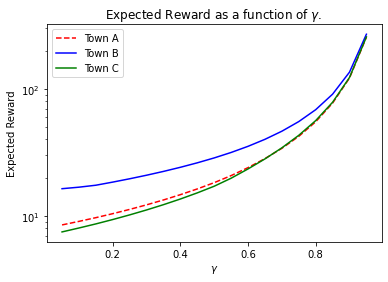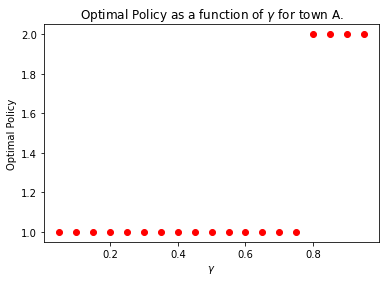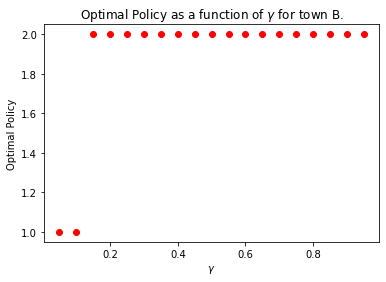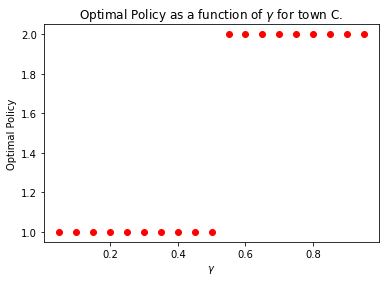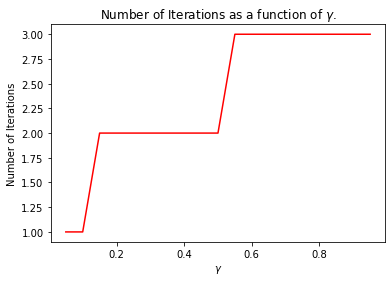In : ## Comparing VI, GSVI import matplotlib.pyplot as plt base_kwargs = check_taxienv() env = TaxiEnv_HW2(**base_kwargs) resultsv, extra_infov = run_value_iteration(env) gamma_values = np.arange(5, 100, 5)/100 iterations = [(extra_infov[i]['iterations']) for i in gamma_values] resultsgv, extra_infogv = run_gauss_seidel_value_iteration(env) iterationsgv = [(extra_infogv[i]['iterations']) for i in gamma_values] plt.figure(5) plt.semilogy(gamma_values,iterations,'r-') plt.semilogy(gamma_values,iterationsgv,'b-') plt.xlabel('$\gamma$') plt.ylabel('Number of Iterations') plt.legend(('Value Iteration','Gauss Seidel Value Iteration')) plt.title('Number of Iterations as a function of$\gamma$.') plt.show()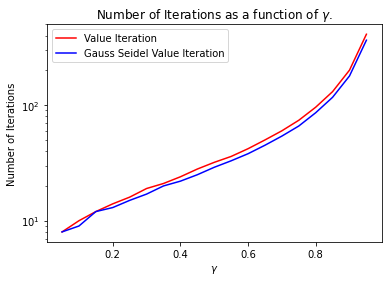# Subjective questions¶ ## 1.a How are values of$\gamma$affecting results of policy iteration¶ It is observed that the expected reward is proportional to the value of$\gamma$and that as gamma becomes closer and closer to 1 the expected reward shoots up. For$\gamma$equal to 1, the expected reward would be infinite. The best policy changed with increasing$\gamma$, going from all 1's to all 2's. This is because when$\gamma$is very small or 0, the algorithm would prefer immediate gains, while if the value of$\gamma$is higher, the algorithm would prefer gains over a longer time period. The number of iterations for Policy iteration also seemed to increase with$\gamma$. ## 1.b For modified policy itetaration, do you find any improvement if you choose m=10.¶ As m increases it is observed that the expected reward also increases, but it does not converge to the actual expected reward as calculated by policy iteration. However, for the example, it was seen that the change of expected reward was quite small as the number of steps increased, and hence cutting off the algorithm early might be a good approximation. ## 1.c Compare and contrast the behavior of Value Iteration and Gauss Seidel Value Iteraton¶ It is observed that both Value Iteration and Gauss Seidel Value Iteration converge to the exact same Expected Reward and Optimal Policy. It is also seen that both the algorithms are by and large the same, since the number of iterations is not too different. The differences are • Value Iteration does not dynamically update values while Gauss Seidel does Dynamically update the values. • Gauss Seidel Value Iteration converged faster than regular Value Iteration for the MDP checked on. • Gauss Seidel Value Iteration does not need an intermediate memory storage of the previous values since it is dynamic. # Submit to AIcrowd 🚀¶ In : !DATASET_PATH=$AICROWD_DATASET_PATH aicrowd notebook submit -c iit-m-rl-assignment-2-taxi -a assets

WARNING: No assets directory at assets... Creating one...
No jupyter lab module found. Using jupyter notebook.
Using notebook: /content/EE17B155_IITM_Assignment_2_Taxi_Release.ipynb for submission...

4/1AY0e-g53yMwSrOcs1n95Bwup0m3uyo2YbQSSHyAUed1RPFqwwK8mY_2EaP0
Mounted at /content/drive
No jupyter lab module found. Using jupyter notebook.
Scrubbing API keys from the notebook...
Collecting notebook...
No jupyter lab module found. Using jupyter notebook.
Validating the submission...
Executing install.ipynb...
[NbConvertApp] Converting notebook /content/submission/install.ipynb to notebook
[NbConvertApp] Executing notebook with kernel: python3
[NbConvertApp] Writing 1033 bytes to /content/submission/install.nbconvert.ipynb
Executing predict.ipynb...
[NbConvertApp] Converting notebook /content/submission/predict.ipynb to notebook
[NbConvertApp] Executing notebook with kernel: python3
[NbConvertApp] ERROR | unhandled iopub msg: colab_request
[NbConvertApp] ERROR | unhandled iopub msg: colab_request
[NbConvertApp] ERROR | unhandled iopub msg: colab_request
[NbConvertApp] ERROR | unhandled iopub msg: colab_request
[NbConvertApp] ERROR | unhandled iopub msg: colab_request
[NbConvertApp] ERROR | unhandled iopub msg: colab_request
[NbConvertApp] Writing 124061 bytes to /content/submission/predict.nbconvert.ipynb
╭───────────────────── Traceback (most recent call last) ──────────────────────╮
│ /usr/local/bin/aicrowd:8 in <module>                                         │
│                                                                              │
│   5 from aicrowd.cli import cli                                              │
│   6 if __name__ == '__main__':                                               │
│   7 │   sys.argv = re.sub(r'(-script\.pyw|\.exe)?\$', '', sys.argv)     │
│ ❱ 8 │   sys.exit(cli())                                                      │
│                                                                              │
│ /usr/local/lib/python3.7/dist-packages/click/core.py:829 in __call__         │
│                                                                              │
│    826 │                                                                     │
│    827 │   def __call__(self, *args, **kwargs):                              │
│    828 │   │   """Alias for :meth:main."""                                 │
│ ❱  829 │   │   return self.main(*args, **kwargs)                             │
│    830                                                                       │
│    831                                                                       │
│    832 class Command(BaseCommand):                                           │
│                                                                              │
│ /usr/local/lib/python3.7/dist-packages/click/core.py:782 in main             │
│                                                                              │
│    779 │   │   try:                                                          │
│    780 │   │   │   try:                                                      │
│    781 │   │   │   │   with self.make_context(prog_name, args, **extra) as c │
│ ❱  782 │   │   │   │   │   rv = self.invoke(ctx)                             │
│    783 │   │   │   │   │   if not standalone_mode:                           │
│    784 │   │   │   │   │   │   return rv                                     │
│    785 │   │   │   │   │   # it's not safe to ctx.exit(rv) here!           │
│                                                                              │
│ /usr/local/lib/python3.7/dist-packages/click/core.py:1259 in invoke          │
│                                                                              │
│   1256 │   │   │   │   Command.invoke(self, ctx)                             │
│   1257 │   │   │   │   sub_ctx = cmd.make_context(cmd_name, args, parent=ctx │
│   1258 │   │   │   │   with sub_ctx:                                         │
│ ❱ 1259 │   │   │   │   │   return _process_result(sub_ctx.command.invoke(sub │
│   1260 │   │                                                                 │
│   1261 │   │   # In chain mode we create the contexts step by step, but afte │
│   1262 │   │   # base command has been invoked.  Because at that point we do │
│                                                                              │
│ /usr/local/lib/python3.7/dist-packages/click/core.py:1259 in invoke          │
│                                                                              │
│   1256 │   │   │   │   Command.invoke(self, ctx)                             │
│   1257 │   │   │   │   sub_ctx = cmd.make_context(cmd_name, args, parent=ctx │
│   1258 │   │   │   │   with sub_ctx:                                         │
│ ❱ 1259 │   │   │   │   │   return _process_result(sub_ctx.command.invoke(sub │
│   1260 │   │                                                                 │
│   1261 │   │   # In chain mode we create the contexts step by step, but afte │
│   1262 │   │   # base command has been invoked.  Because at that point we do │
│                                                                              │
│ /usr/local/lib/python3.7/dist-packages/click/core.py:1066 in invoke          │
│                                                                              │
│   1063 │   │   """                                                           │
│   1064 │   │   _maybe_show_deprecated_notice(self)                           │
│   1065 │   │   if self.callback is not None:                                 │
│ ❱ 1066 │   │   │   return ctx.invoke(self.callback, **ctx.params)            │
│   1067                                                                       │
│   1068                                                                       │
│   1069 class MultiCommand(Command):                                          │
│                                                                              │
│ /usr/local/lib/python3.7/dist-packages/click/core.py:610 in invoke           │
│                                                                              │
│    607 │   │   args = args[2:]                                               │
│    608 │   │   with augment_usage_errors(self):                              │
│    609 │   │   │   with ctx:                                                 │
│ ❱  610 │   │   │   │   return callback(*args, **kwargs)                      │
│    611 │                                                                     │
│    612 │   def forward(*args, **kwargs):  # noqa: B902                       │
│    613 │   │   """Similar to :meth:invoke but fills in default keyword     │
│                                                                              │
│ /usr/local/lib/python3.7/dist-packages/aicrowd/cmd/notebook.py:92 in         │
│ submit_subcommand                                                            │
│                                                                              │
│    89 │   │   output,                                                        │
│    90 │   │   notebook_name,                                                 │
│    91 │   │   no_verify,                                                     │
│ ❱  92 │   │   dry_run,                                                       │
│    93 │   )                                                                  │
│                                                                              │
│ /usr/local/lib/python3.7/dist-packages/aicrowd/notebook/submit.py:94 in      │
│ create_submission                                                            │
│                                                                              │
│   91 │   │   │   challenge=challenge,                                        │
│   92 │   │   │   file_path=submission_zip_path,                              │
│   93 │   │   │   description=description,                                    │
│ ❱ 94 │   │   │   print_links=True,                                           │
│   95 │   │   )                                                               │
│                                                                              │
│ /usr/local/lib/python3.7/dist-packages/aicrowd/submission/create_cmd.py:90   │
│ in create                                                                    │
│                                                                              │
│   87 │   if file_path is None:                                               │
│   88 │   │   raise NotImplementedError("Git based submissions are not ready  │
│   89 │                                                                       │
│ ❱ 90 │   return submit_file(challenge_slug, file_path, description, api_key, │
│                                                                              │
│ /usr/local/lib/python3.7/dist-packages/aicrowd/submission/helpers.py:346 in  │
│ submit_file                                                                  │
│                                                                              │
│   343 │   │   │   "Error in getting presigned url for s3 upload: %s",        │
│   344 │   │   │   s3_presigned_details.get("message"),                       │
│   345 │   │   )                                                              │
│ ❱ 346 │   │   raise SubmissionUploadException(s3_presigned_details.get("mess │
│   347 │                                                                      │
│   348 │   s3_key = upload_submission(                                        │
│   349 │   │   s3_presigned_details["url"], s3_presigned_details["fields"], f │
╰──────────────────────────────────────────────────────────────────────────────╯